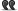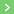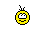Topogical properties of the set of algebraic numbers

# Topogical properties of the set of algebraic numbers

1. ## Topogical properties of the set of algebraic numbers

------

Hello,

Question is: Modulus or Module?

Context comes from a French book and concerns the construction of "the number system and (structural) topological properties".

(1). Set of natural numbers -> (2). Set of integers -> (3). Set of rational numbers -> [(4). Set of real numbers , (5). Set of algebraic numbers] -> (6). Set of complex numbers

Consider the "topological properties" of such sets:

(3). Topological property of the "Set of rational numbers" is: "Non complete metric space for the topology of the absolute value".

(4). Topological property of the "Set of real numbers" is: "Complete metric space".

(5). Topological property of the "Set of algebraic numbers" is: "Non complete metric space for the topology of the modulus". (Is it Module or Modulus here?)

(6). Topological property of the "Set of complex numbers" is: "Complete metric space (any Cauchy sequence converges)".

*In french module is used to express both English terms Modulus and Module, so to translate the text from french to english I meet a problem. Furthermore, the term "Module" regarded as a "vector space" in which the scalar is a ring rather a field, is used in particular in Abstract algebra , Homology theory and Extension field.

I share my doubt with you, is it Modulus or Module?

(In spite of what precedes:*) I think it could be rather "Modulus" referring to the metric notion.

Is there someone to help me to resolve this question?
Thank you so much.

-----2. ## Re : Topogical properties of the set of algebraic numbers

I do believe it is "Module", the complex equivalent of the the "absolute value".3. ## Re : Topogical properties of the set of algebraic numbers

Salut.

The absolute value on the set of real numbers is still called "absolute value" in English, when extended to the set of complex numbers. But the term "modulus" is a synonym :

Wikipedia (En)

In French, the situation is different, as we usually use the word "module" for that notion (and also in the context of "modules over a ring" = "modules sur un anneau", so I agree with you, it's ambiguous), and prefer to say "valeur absolue" only in the case of real or p-adic numbers.

Taar.4. ## Re : Topogical properties of the set of algebraic numbers

Thanks. The context and remarks comes from a French book in which unfortunately there is no comment. Such an approach seems very interesting, but a doubt appears to me about this question. I think it is modulus rather than module, but doubt persists.5. ## Re : Topogical properties of the set of algebraic numbersEnvoyé par MédiatI do believe it is "Module", the complex equivalent of the the "absolute value".

Math Oxford dictionary says: "Modulus of complex number". It's why I would tend to use rather modulus. What do you think?6. ## Re : Topogical properties of the set of algebraic numbersEnvoyé par th..Math Oxford dictionary says: "Modulus of complex number". It's why I would tend to use rather modulus. What do you think?
Undoubtedly, my English is a bit less accurate than the Math Oxford dictionarys one, so I have to admit it is modulus.
The important part is that it is the complex equivalent of the "absolute value".7. ## Re : Topogical properties of the set of algebraic numbersEnvoyé par MédiatUndoubtedly, my English is a bit less accurate than the Math Oxford dictionarys one, so I have to admit it is modulus.
The important part is that it is the complex equivalent of the "absolute value".
Thanks. Th.8. ## Re : Topogical properties of the set of algebraic numbers

So, is there someone who can decide between Modulus and module within such a framework?9. ## Re : Topogical properties of the set of algebraic numbers

Salut.Envoyé par th..So, is there someone who can decide between Modulus and module within such a framework?
Mediat and I actually just did this.

It's "modulus".

Taar.10. ## Re : Topogical properties of the set of algebraic numbersEnvoyé par TaarSalut.

Mediat and I actually just did this.

It's "modulus".

Taar.
Thanks Taar and Mediat. Th.